Courses

# Chapter 5 - Polyphase Synchronous Machines (Part - 1) - notes of Electrical Engineering Electrical Engineering (EE) Notes | EduRev

## Electrical Engineering (EE) : Chapter 5 - Polyphase Synchronous Machines (Part - 1) - notes of Electrical Engineering Electrical Engineering (EE) Notes | EduRev

The document Chapter 5 - Polyphase Synchronous Machines (Part - 1) - notes of Electrical Engineering Electrical Engineering (EE) Notes | EduRev is a part of the Electrical Engineering (EE) Course Electrical Engineering SSC JE (Technical).
All you need of Electrical Engineering (EE) at this link: Electrical Engineering (EE)

Introduction
• Rotating machines that rotate at a speed fixed by the supply frequency and the number of poles are called synchronous machines.
• Three phase synchronous machine is a doubly excited ac machine its field-winding is energized from a dc source i.d. DC exciters, static excitation etc. and Armature winding is connected to an ac source.
• Under steady state conditions, operating speed of synchronous machine depends on the frequency of armature current and number of field poles.
• Synchronous speed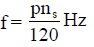Where P = No. of fields poles
ns = rotor speed in rps (called synchronous speed)
f = frequency of armature current

Remember:
• In synchronous machine 3 - ø armature winding is on stator and field winding on rotor.

Constructional Features

• In synchronous machines, the armature winding either exports ac power (synchronous generator) or imports ac power (synchronous motor), whereas the field winding is always from a dc source.
• An ac generator, alternator or synchronous generator may have either rotating field poles and stationary armature, or rotating armature and stationary field poles.
• Advantage of providing the field winding on rotor and armature winding on the stator:
• More Efficient: With armature winding on the stator and field winding on the rotor only two slip rings are required in a synchronous machine.
• Better Insulation: Stationary armature windings can be insulated satisfactorily for higher voltages.
• Efficient Cooling: Stationary armature winding can be cooled more efficiently.
• More Output : Low-power field winding on the rotor gives a lighter rotor and therefore, low centrifugal forces. In view of this, higher rotor speeds are permissible, thus increasing the synchronous machine output for given dimensions.
• Lesser Rotor Weight Inertia: Field winding on the rotor requires less amount of copper and insulation. This reduces overall weight of rotor and its inertia.
• Rigid and Convenient Construction: 3 - ø armature winding, capable of handling high voltage and high current, can be more easily braced again electromagnetic forces when it is placed in stator slots.
• Synchronous machines are of two types depending upon the geometrical structure of the rotor:
• salient-pole type.
• cylindrical-rotor, round-rotor or non-salient pole type.

Remember:
• The field winding on the salient poles is a concentrated winding.
• In case of cylindrical rotor, the field winding is a distributed winding housed in the rotor slots.
• The salient pole synchronous machines have non-uniform air gap.

• Under the pole centres it is minimum
• In between the poles, the air-gap it is minimum

• In cylindrical rotor synchronous machine, the air-gap is uniform throughout, neglecting the slot-openings.
• Synchronous generators are usually of 3 - ø  type because of the several advantage associated with 3 - ø  generation, transmission and high-power utilization.

• For the generation of 3 - ø voltage, at least three coils (one coil per phase), phase displaced by 120 electrical degrees in space, are required.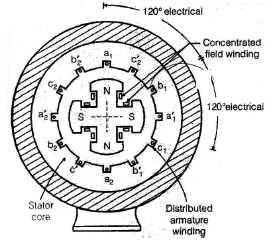• Oil engines to fewer number of poles, say 2 or 4.

Generated EMF
In this type of machines, air-gap flux is constant in amplitude.

Armature winding
• The generated emf in any one phase of a synchronous machine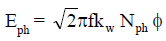• In a rotating machine, the relative motion between armature coil and flux-density wave, causes flux linking the coil to vary with time and as a result, an emf is induced in the armature coil.
• Flux ø is the total flux per pole.
• The armature winding is distributed and the reduction factor kw must appear in the emf expression.

The Field Winding

• The field winding of a synchronous machine is always energized with direct current. Under steady state conditions, the field or exciting current.

If = V/rf

Where
V= Direct voltage applied to the field winding

and rf = Field winding resistance

FLUX AND MMF PHASORS

Cylindrical Rotor Synchronous Machines
• The alternator terminal voltage at no load is made equal to its rated value by adjusting its field current.
• Generated emf lags by 90º the flux that generates it. This is indicated in Figure, where Ef is shown lagging øf by 90º.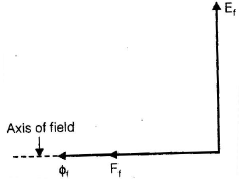• Field mmf per pole Ff is equal to If Nf . As saturation is ignored, field flux phasor øf is also indicated in phase with field mmf Ff.

• The emf generated by øf alone is called the excitation voltage.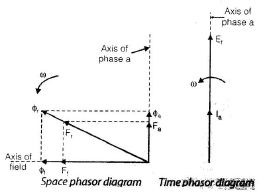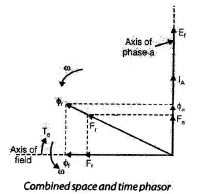• When alternator is connected to 3-phase load, 3-phase generated emf in armature will give rise to 3-phase balanced currents.
• Unity pf means that armature current Ia and excitation voltage are maximum at the same instant of time.
• The mmf set up by armature current is called the armature-reaction mmf.
• For balanced poly-phase currents flowing in poly-phase winding, the peak value of the resultant mmf wave is along that phase-axis which carries the maximum current.
• The phasor sum Fand Fa gives the resultant air gap mmf Fr.
• For generator operation, the prim mover torque must be opposite to this electromagnetic torque.
• Armature rotating mmf Fa, given by equation, is proportional to armature current Ia and is therefore in phase with Ia.
• Armature mmf Fa is perpendicular to field flux øf therefore armature reaction mmf at unity pf is cross-magnetizing in nature.

Case - 3: Zero pf Lagging Load
• Flux created by armature mmf Fa directly opposes the field mmf Ff.
• Ef lags øby 90º, Ia lags Ef by 90º. Therefore Flags øor Ff by 180º, i.e. Fa oppose field mmf Ff.
• For zero pf lagging load on the 3-phase alternator, the nature of armature mmf is entirely demagnetizing in nature.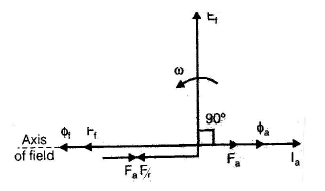• Ef is shown lagging øf by 90º, Ia leads Ef by 90º and Fa is in phase with Ff so that resultant mmf F= algebraic sum of Ff and Fa.
• Flux created by armature mmf Fa directly aids the field mmf For the field flux øf .

• For zero pf leading load on a 3-phase alternator, the armature mmf is entirely magnetizing in nature.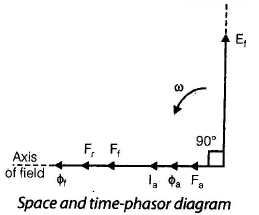Case 5 : Lagging pf Load
• Let us consider a general case of armature current Ilagging the excitation voltage by a time-phase angle ψº electrical. This means that load pf with respect to Ef is cos ψº lagging.
• Armature reaction mmf Fa lags behind the field mmf Ff by a space angle of (90+ ψº). Resultant of mmfs Ff and Fa gives mmf Fr.
• Ilags Ef by ψº because load pf is cose ψ lagging.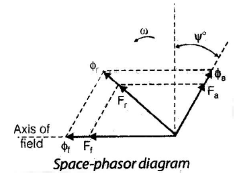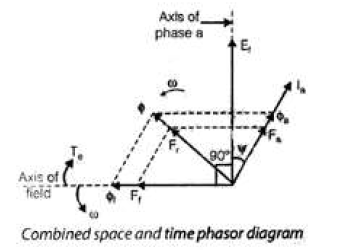Cylindrical-rotor Synchronous Motor
• Rotating armature mmf phasor Fa is in phase with Ia. Armature-reaction flux øa is also in phase with Fa.
• The resultant mmf Fr is obtained by the phasor sum of Fand Ff, i.e.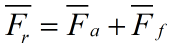• For motor operation, the field poles must be dragged behind the resultant air-gap flux by the retarding shaft-load torque.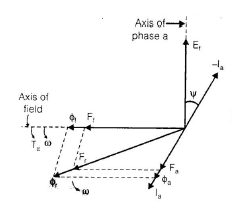Combined space and time phasor diagram with Ia lagging Ef
• When Ia lags Ef by 90º, armature current Ia lags the excitation emf Ef by 90º, the nature of armature mmf, or armature reaction mmf Fa is

• Magnetizing in case of alternator and
• Demagnetizing in a synchronous motor.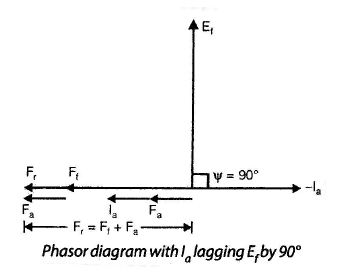Cylindrical Rotor Alternator

• The flux actually existing in the air-gap of a machine is due to the resultant mmf of all the windings. The field mmf Fand armature reaction mmf Fa have been combined together to give the resultant mmf Fr, in a cylindrical-rotor synchronous machine.
• The phasor addition of the two mmfs Fand Fa is possible because of the fact that:
• These two mmfs are distributed sinusoidally along the air gap periphery and
• the relative velocity between the two mmfs is zero at synchronous speed, i.e. the stator and rotor mmfs are stationary with respect to each other.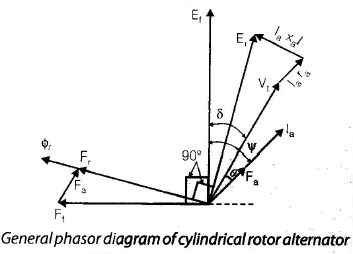Note:
• These are useful for finding out the parameters of the synchronous machines and determine their performance.
• For obtaining the open-circuit characteristic (OCC), the alternator is driven at constant rated speed and the open circuit terminal voltage is
noted as the field current is gradually increased from zero.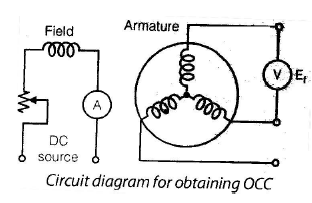• The OCC is a graph between the field current If or field mmf Ff and the generated emf Ef.
• At small value of field current or Ff, the air gap requires almost the whole of Ff and mmf required by the iron is almost negligible. But when the mmf has exceeded a certain value, the iron parts require a good amount of mmf and the saturation sets in.

Short Circuit Characteristics
• For obtaining the short-circuit characteristics, the machine is driven at rated synchronous speed and the armature terminals are shot-circuited through an ammeter.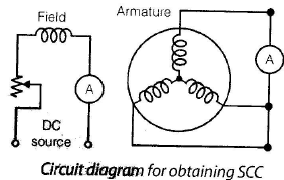• Alternator during SC test operates under unsaturated conditions and as a result SCC is a straight line.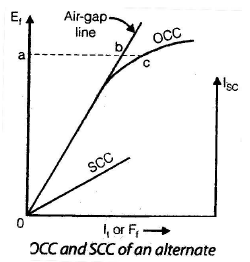Zero Power-Factor Characteristic and Potier Triangle
• z.p.f.c. of an alternator is a plot between armature terminal voltage and its field current for constant values of armature current and speed.
• z.p.f.c. in conjugation with OCC, is useful in obtaining the armature leakage reactance xal and armature reaction mmf Fa.
• For an alternator, zero-power-facto characteristic is obtained as follow:

• The synchronous machine is run at rated synchronous speed by the prime-mover
• A purely inductive load is connected across the armature terminals and field current is increased till full load armature current is flowing.
• The load is varied in steps and the field current at each step is adjusted to maintain full-load armature current. The plot of armature terminal voltage and field current recorded at each step, gives the zero-power factor characteristic at full load armature current.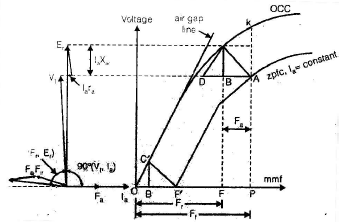• From this figure the terminal voltage V1 and the air gap voltage Er, are very nearly in phase
Vt = Er – IaxaI
• The resultant m.m.f. Fr and the field m.m.f. F1 are also related by the simple algebraic equation

Voltage Regulation of an Alternator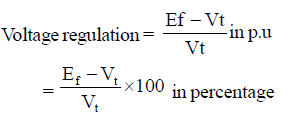• Here E1 is the no-load excitation voltage and Vis full-load terminal voltage at the same speed and field excitation.
• In large machines, it may not be possible to obtain the voltage regulation by actual loading, because of the cost of dissipating the huge output and also providing the large input. Certain simple tests, involving only small amounts of power, are conducted and from these, the machine constants are determined to compute the voltage regulation

Methods for Computing Voltage Regulation
Electromotive Force (emf) Method
• Also known as synchronous impedance method
• This method can be applied to cylindrical rotor synchronous machines only, because the resultant air-gap flux ør is not affected by the angular position of the rotor.

Assumptions:
• The iron par of the magnetic circuit is have constant permeability.
• As the saturation is neglected the mmf can be replaced by their corresponding fluxes and therefore, the corresponding emfs.

• Field mmf Ff generates Elagging it by 90º, resultant mmf Fr generates air-gap voltage Elagging it by 90º, similarly armature reaction mmf Fa must generate armature reactor emf Ear lagging Fa by 90º.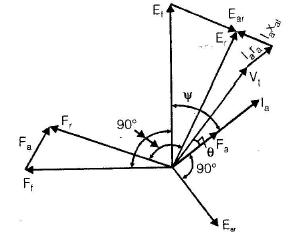• From above figure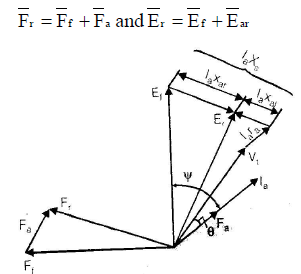• From above figure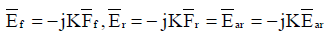Here K is the slope of the air-gap line

• The armature -reaction mmf Fa- is in phase with, and proportional to, armature current la.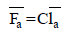where C is a constant.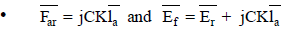• Phasor sum of Vt, la ra and la ×al gives air gap emf Er.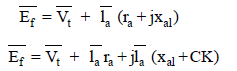• As CK has the dimension of a reactance we can assume.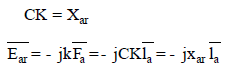• Reactance Xar is due to the presence of armature reactions mmf Fa.
• The total equivalent reactance
Xal + Xar = Xs
Where Xs is called Synchronous reactance of the cylindrical- rotor synchronous machine.
• The reactance Xar, due to armature reaction mmf is called armature reaction reactance or magnetizing reactance.

NOTE:-
• Xai is a fictitious reactance and it accounts for the voltage Ear generated by armature reaction mmf Fa.
• The term (ra + jXs) = Zs, is called the synchronous impedance of the cylindrical- rotor synchronous machine.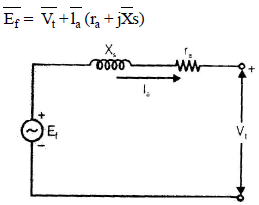Equivalent circuit for a cylindrical rotor synchronous generator
Remember :-
• for an alternator, the power and la flows out of the machine. For a Synchronous motor, the power and la flows into the machine.
• The synchronous motor voltage equation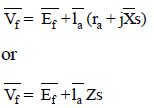Measurement of Zs and Xs
• Open-circuit and short circuit characteristics are required for the determination of Zs and Xs.

• In open-circuit test, the armature current la is zero and V= Ef.
• In the short-circuit test, entire emf Ef is consumed in circulating the short-circuit current lsc, through the synchronous impedance Z .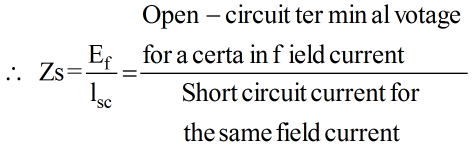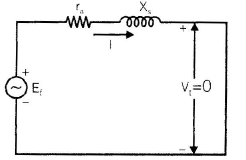Equivalent circuit under short circuit test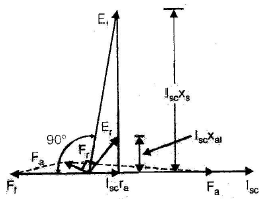Phasor diagram under short circuit test

NOTE:
• If there were no saturation, Zs would be constant. Actually Zs is variable and it decreases with the onset of saturation in the OCC.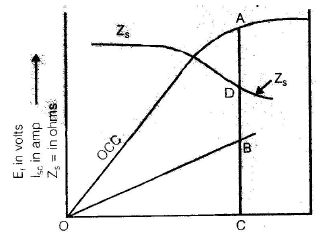Determination of synchronous impedance of an alternator
• For calculating the voltage regulation, only one value of Zs can be used the lowest value of Zs, obtained from the largest possible short-circuit current, is used for determining the voltage regulation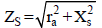• The dc resistance rdc of one phase is measured, by voltmeter ammeter method.
• If the armature winding is star connected and neutral is not available, then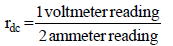• In case the armature winding is delta-connected, then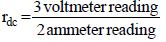• The effective armature resistance per phase

ra = (1.2 to 1.3)rdc

• After ra is calculated , Xs can be determined.
Voltage phasor Diagram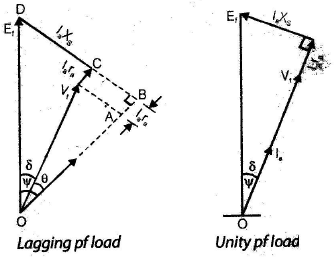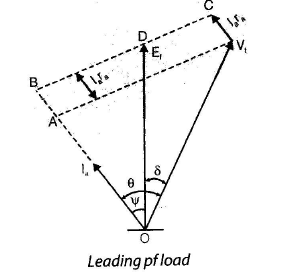• For laggig power factor load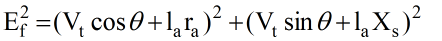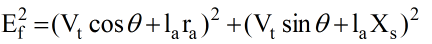• For unity power factor load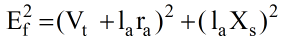• Now the voltage regulation in percentage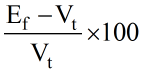NOTE:-
• As unsaturated value of Zs is more than the saturated value, voltage regulation computed by emf method is much higher than the actual value. It is because of this reason that the emf method is called pessimistic method.
The Magnetomotive Force (mmf) method

Assumption:
• Each emf is replaced by an equivalent mmf (uniform air-gap and neglect of saturation.)
• The voltage equation of a synchronous machine, working as an alternator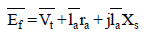Division of above equation by -jK converts it into an mmf equation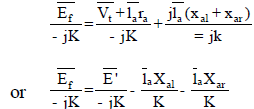•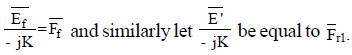The field mmf Finduces, in the armature winding, an emf Ef lagging it by 90º, similarly the mmf Fr must induce an emf E' lagging Fr1 by 90º.

•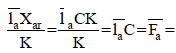armature reaction mmf in phase with la. Here armature reaction reactance drop la Xar, has been transformed into mmf Fa.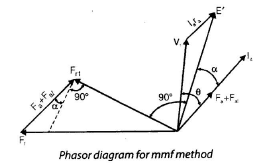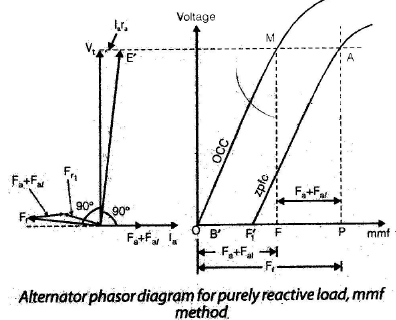• From above figure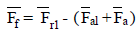NOTE:-
• The mmf (Fal- +Fa ) is in phase with the armature current la.

• α is the angle (i) by which llags E' and (ii) between the normal line of Fr1 and (Fa + Fal).
• To obtain voltage regulation by mmf method

1. Plot OCC and SCC
2. find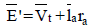and obtain the corresponding value of Fr1 from OCC
3. Find (Fa + Fal) from SCC
4. Calculate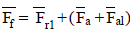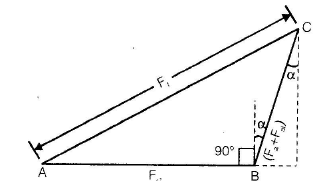• AC gives the required value of field mmf Ff which is given by the relation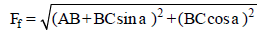corresponding to field mm Ff , obtain Ef from OCC and thus the voltage regulation of the alternator.

Zero Power factor Method
• The emfs are handled as voltage and the mmfs as field ampere-turns of field amperes.
• The armature reaction mmf Fa and armature leakage reactance Xal , can be determined from the potier triangle, as explained before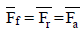is obtained and corresponding to Ff . excitation voltage Ef is recorded from OCC and the voltage regulation obtained.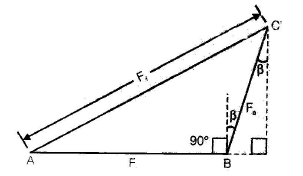Remember:
• Zpf method required OCC and zpfc, and gives quite accurate results.
New ASA (American standards association) method

NOTE:
• This method is essentially a modification of the mmf method and gives satisfactory results both for cylindrical rotor and salient pole synchronous machines.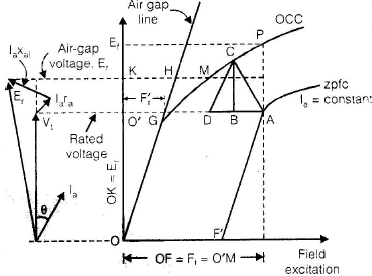• New ASA method requires OCC and zpfc. Only two points A and F', are sufficient to be known on the zpfc.
• The point A is obtained by loading the overexcited alternator by an underexcited synchronous motor till full load armature current at rated voltage is flowing.
• The point F' is obtained by noting field excitation (fa + fal ), required to circulate full load armature current when the alternator is short-circuited.
• The armature leakage reactance Xal is determined from the potier reactance drop BC.

• Now determine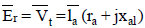and use the magnitude of Ein obtaining the saturation effects.
Ok = E. This line intersects the air gap line at H and the OCC at M. The distance HM, On the field excitation scale, gives the additional excitation that must be added to the unsaturated excitation O'H, to determine the total excitation O'M = F.
• Corresponding to O'M = F= OF, excitation voltage FP = EF is read from OCC and the voltage regulation obtained.

Saturation Synchronous Reactance Method
• In emf and mmf methods, the saturation was neglected. But under actual operating conditions, the magnetic circuit is always in a saturated state.
• The extent of this saturation under load, can be taken into account by introducing saturation factor k.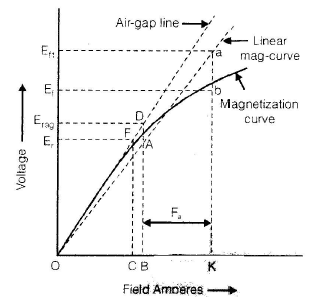• The saturation factor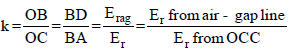for the same field mmf or field current.

• the synchronous reactance XS has two components, Xal and xav the leakage reactance Xal remains constant, because the leakage flux path is mainly is air and is almost unaffected by saturation.
• The armature reaction reactance Xar is affected by the magnetic saturation because the path of the armature reaction flux is mainly through iron.
• The saturation factor k, should be applied to the magnetizing reactance Xar only.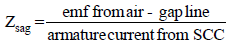for the same field current.

Remember:-
• The unsaturated synchronous impedance Zsag remains constant and may be calculated for any value of field current.
• Unsaturated synchronous reactance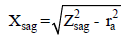• Armature reaction reactance
Xar =Xsag - Xal.
• The saturated synchronous reactance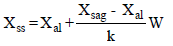• In order to use the saturated synchronous reactance method:

• First calculate air- gap voltage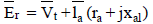• Mark Er = BA on the OCC and find the corresponding voltage BD, on the air-gap line.
• Obtain the saturation factor as K = BD/BA
• Calculate Xsag
• Find saturation synchronous reactance Xss
• Draw phasor diagram with Xs replaced by Xss – now calculate Ef and thus the voltage regulation.
Offer running on EduRev: Apply code STAYHOME200 to get INR 200 off on our premium plan EduRev Infinity!

65 docs|37 tests

,

,

,

,

,

,

,

,

,

,

,

,

,

,

,

,

,

,

,

,

,

;